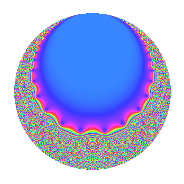Properties

 Label 8.12.a.bLevel 8 Weight 12 Character orbit 8.a Self dual Yes Analytic conductor 6.147 Analytic rank 0 Dimension 2 CM No Inner twists 1

Related objects

Newspace parameters

 Level: $$N$$ = $$8 = 2^{3}$$ Weight: $$k$$ = $$12$$ Character orbit: $$[\chi]$$ = 8.a (trivial)

Newform invariants

 Self dual: Yes Analytic conductor: $$6.14674544448$$ Analytic rank: $$0$$ Dimension: $$2$$ Coefficient field: $$\Q(\sqrt{109})$$ Coefficient ring: $$\Z[a_1, a_2, a_3]$$ Coefficient ring index: $$2^{7}$$ Fricke sign: $$1$$ Sato-Tate group: $\mathrm{SU}(2)$

$q$-expansion

Coefficients of the $$q$$-expansion are expressed in terms of $$\beta = 64\sqrt{109}$$. We also show the integral $$q$$-expansion of the trace form.

 $$f(q)$$ $$=$$ $$q$$ $$+ ( 28 + \beta ) q^{3}$$ $$+ ( 3934 - 12 \beta ) q^{5}$$ $$+ ( 45528 + 42 \beta ) q^{7}$$ $$+ ( 270101 + 56 \beta ) q^{9}$$ $$+O(q^{10})$$ $$q$$ $$+ ( 28 + \beta ) q^{3}$$ $$+ ( 3934 - 12 \beta ) q^{5}$$ $$+ ( 45528 + 42 \beta ) q^{7}$$ $$+ ( 270101 + 56 \beta ) q^{9}$$ $$+ ( 79540 - 693 \beta ) q^{11}$$ $$+ ( 525238 + 948 \beta ) q^{13}$$ $$+ ( -5247416 + 3598 \beta ) q^{15}$$ $$+ ( 715442 - 10824 \beta ) q^{17}$$ $$+ ( -10933300 - 4587 \beta ) q^{19}$$ $$+ ( 20026272 + 46704 \beta ) q^{21}$$ $$+ ( 17903368 - 24738 \beta ) q^{23}$$ $$+ ( 30939047 - 94416 \beta ) q^{25}$$ $$+ ( 27604696 + 94522 \beta ) q^{27}$$ $$+ ( -114413850 + 59556 \beta ) q^{29}$$ $$+ ( 32361056 - 28632 \beta ) q^{31}$$ $$+ ( -307172432 + 60136 \beta ) q^{33}$$ $$+ ( -45910704 - 381108 \beta ) q^{35}$$ $$+ ( 37779390 - 81564 \beta ) q^{37}$$ $$+ ( 437954536 + 551782 \beta ) q^{39}$$ $$+ ( 600607098 + 647088 \beta ) q^{41}$$ $$+ ( -22759916 + 324051 \beta ) q^{43}$$ $$+ ( 762553526 - 3020908 \beta ) q^{45}$$ $$+ ( -614539632 - 816420 \beta ) q^{47}$$ $$+ ( 883034537 + 3824352 \beta ) q^{49}$$ $$+ ( -4812493960 + 412370 \beta ) q^{51}$$ $$+ ( -1904274962 + 3514980 \beta ) q^{53}$$ $$+ ( 4025704984 - 3680742 \beta ) q^{55}$$ $$+ ( -2354062768 - 11061736 \beta ) q^{57}$$ $$+ ( -3006463292 + 4307463 \beta ) q^{59}$$ $$+ ( 4894896454 + 4588692 \beta ) q^{61}$$ $$+ ( 13347241656 + 13893810 \beta ) q^{63}$$ $$+ ( -3012688172 - 2573424 \beta ) q^{65}$$ $$+ ( 7351547612 - 21317583 \beta ) q^{67}$$ $$+ ( -10543332128 + 17210704 \beta ) q^{69}$$ $$+ ( 2159995544 - 20099142 \beta ) q^{71}$$ $$+ ( 5527819738 - 3259368 \beta ) q^{73}$$ $$+ ( -41287051708 + 28295399 \beta ) q^{75}$$ $$+ ( -9373484064 - 28210224 \beta ) q^{77}$$ $$+ ( 25978811632 + 41373444 \beta ) q^{79}$$ $$+ ( -4873980151 + 20331080 \beta ) q^{81}$$ $$+ ( -54113987956 - 13552251 \beta ) q^{83}$$ $$+ ( 60804864860 - 51166920 \beta ) q^{85}$$ $$+ ( 23386022184 - 112746282 \beta ) q^{87}$$ $$+ ( 35594145930 + 90758808 \beta ) q^{89}$$ $$+ ( 41689446288 + 65220540 \beta ) q^{91}$$ $$+ ( -11877047680 + 31559360 \beta ) q^{93}$$ $$+ ( -18436437784 + 113154342 \beta ) q^{95}$$ $$+ ( -849903838 - 64199400 \beta ) q^{97}$$ $$+ ( 4157458628 - 182725753 \beta ) q^{99}$$ $$+O(q^{100})$$ $$\operatorname{Tr}(f)(q)$$ $$=$$ $$2q$$ $$\mathstrut +\mathstrut 56q^{3}$$ $$\mathstrut +\mathstrut 7868q^{5}$$ $$\mathstrut +\mathstrut 91056q^{7}$$ $$\mathstrut +\mathstrut 540202q^{9}$$ $$\mathstrut +\mathstrut O(q^{10})$$ $$2q$$ $$\mathstrut +\mathstrut 56q^{3}$$ $$\mathstrut +\mathstrut 7868q^{5}$$ $$\mathstrut +\mathstrut 91056q^{7}$$ $$\mathstrut +\mathstrut 540202q^{9}$$ $$\mathstrut +\mathstrut 159080q^{11}$$ $$\mathstrut +\mathstrut 1050476q^{13}$$ $$\mathstrut -\mathstrut 10494832q^{15}$$ $$\mathstrut +\mathstrut 1430884q^{17}$$ $$\mathstrut -\mathstrut 21866600q^{19}$$ $$\mathstrut +\mathstrut 40052544q^{21}$$ $$\mathstrut +\mathstrut 35806736q^{23}$$ $$\mathstrut +\mathstrut 61878094q^{25}$$ $$\mathstrut +\mathstrut 55209392q^{27}$$ $$\mathstrut -\mathstrut 228827700q^{29}$$ $$\mathstrut +\mathstrut 64722112q^{31}$$ $$\mathstrut -\mathstrut 614344864q^{33}$$ $$\mathstrut -\mathstrut 91821408q^{35}$$ $$\mathstrut +\mathstrut 75558780q^{37}$$ $$\mathstrut +\mathstrut 875909072q^{39}$$ $$\mathstrut +\mathstrut 1201214196q^{41}$$ $$\mathstrut -\mathstrut 45519832q^{43}$$ $$\mathstrut +\mathstrut 1525107052q^{45}$$ $$\mathstrut -\mathstrut 1229079264q^{47}$$ $$\mathstrut +\mathstrut 1766069074q^{49}$$ $$\mathstrut -\mathstrut 9624987920q^{51}$$ $$\mathstrut -\mathstrut 3808549924q^{53}$$ $$\mathstrut +\mathstrut 8051409968q^{55}$$ $$\mathstrut -\mathstrut 4708125536q^{57}$$ $$\mathstrut -\mathstrut 6012926584q^{59}$$ $$\mathstrut +\mathstrut 9789792908q^{61}$$ $$\mathstrut +\mathstrut 26694483312q^{63}$$ $$\mathstrut -\mathstrut 6025376344q^{65}$$ $$\mathstrut +\mathstrut 14703095224q^{67}$$ $$\mathstrut -\mathstrut 21086664256q^{69}$$ $$\mathstrut +\mathstrut 4319991088q^{71}$$ $$\mathstrut +\mathstrut 11055639476q^{73}$$ $$\mathstrut -\mathstrut 82574103416q^{75}$$ $$\mathstrut -\mathstrut 18746968128q^{77}$$ $$\mathstrut +\mathstrut 51957623264q^{79}$$ $$\mathstrut -\mathstrut 9747960302q^{81}$$ $$\mathstrut -\mathstrut 108227975912q^{83}$$ $$\mathstrut +\mathstrut 121609729720q^{85}$$ $$\mathstrut +\mathstrut 46772044368q^{87}$$ $$\mathstrut +\mathstrut 71188291860q^{89}$$ $$\mathstrut +\mathstrut 83378892576q^{91}$$ $$\mathstrut -\mathstrut 23754095360q^{93}$$ $$\mathstrut -\mathstrut 36872875568q^{95}$$ $$\mathstrut -\mathstrut 1699807676q^{97}$$ $$\mathstrut +\mathstrut 8314917256q^{99}$$ $$\mathstrut +\mathstrut O(q^{100})$$

Embeddings

For each embedding $$\iota_m$$ of the coefficient field, the values $$\iota_m(a_n)$$ are shown below.

For more information on an embedded modular form you can click on its label.

Label $$\iota_m(\nu)$$ $$a_{2}$$ $$a_{3}$$ $$a_{4}$$ $$a_{5}$$ $$a_{6}$$ $$a_{7}$$ $$a_{8}$$ $$a_{9}$$ $$a_{10}$$
1.1
 −4.72015 5.72015
0 −640.180 0 11952.2 0 17464.5 0 232683. 0
1.2 0 696.180 0 −4084.16 0 73591.5 0 307519. 0
 $$n$$: e.g. 2-40 or 990-1000 Significant digits: Format: Complex embeddings Normalized embeddings Satake parameters Satake angles

Inner twists

This newform does not admit any (nontrivial) inner twists.

Atkin-Lehner signs

$$p$$ Sign
$$2$$ $$1$$

Hecke kernels

This newform can be constructed as the kernel of the linear operator $$T_{3}^{2}$$ $$\mathstrut -\mathstrut 56 T_{3}$$ $$\mathstrut -\mathstrut 445680$$ acting on $$S_{12}^{\mathrm{new}}(\Gamma_0(8))$$.